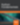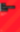# Ruby Interview Questions: LRU Cache and Binary Trees

Ruby

Share:

#### Free JavaScript Book!Write powerful, clean and maintainable JavaScript.

RRP \$11.95As we talked about last time around, most interview problems for software engineering positions across the industry concern algorithms and data structures. With a bit of practice, it is possible and reasonably enjoyable to tackle these problems in Ruby. We have covered some of the basics: linked lists and hash tables. This time, we’ll go a bit deeper. We’ll implement an LRU cache (and explain what that is) and also look into binary trees, all in Ruby.

## LRU Cache

Say we want to build a cache of items and that this cache can only hold a fixed number of things. This begs the question: what happens when the cache is full and we want to insert an item? A common way to answer this question is with the Least Recently Used (LRU) eviction policy. Basically, it means that we remove (i.e. evict) the least recently used item in the cache to make room for the new item.

The question is this: how do we implement an efficient LRU cache? The cache has to be able to do a couple of things. First, we must be able to insert things into it with keys associated with them. Second, we should be able to get an item using its key.

This is an incredibly common interview question and one that is still asked in initial rounds. It requires the combination of a couple of concepts to get right. The LRU cache is most often implemented as a hash table and a doubly linked list (you can also use a splay tree, but that’s less common). Here’s how it works. The hash table’s keys consist of the keys for our cache and values are actually nodes within the doubly linked list. We always keep track of the head and tail of this list. When we want to get a value out this cache, we look for that key in the hash table and return the associated node from the doubly linked list. In addition, we move that node from wherever it is in the list to the very back of the list. This makes sure that the least recently used item is always at the very front of the list. So, when we want to insert a new item into our cache and the cache is full, we simply pop off an item from the front of the list.

To implement this in Ruby, we need a doubly linked list. Thankfully, this is pretty easy to implement:

``````class Node
attr_accessor :value, :next_node, :prev_node

def initialize(value, prev_node, next_node)
@value = value
@prev_node = prev_node
@next_node = next_node
end
end
``````

We’re just keeping track of the next node and previous node. Now, onto implementing our cache. As mentioned, we need to keep track of a couple of things: the head of the list, the tail of the list and the number of items in the cache.

``````  class LRU
def initialize(max_items)
@max_items = max_items
@table = {}
@tail = nil
@num_items = 0
end
end
``````

Now, let’s figure out how to implement the `set(key, value)` method.

``````class LRU
...
def set(key, value)
@num_items += 1
if @num_items > @max_items
end

new_node = Node.new value, @tail, nil
@head = new_node if tail == nil
@tail.next_node = new_node if @tail != nil
@tail = new_node
@table[key] = new_node
end
...
end
``````

The code is fairly straightforward if we step through it. First, we check if the cache is full. If so, we have to evict from the head of the doubly linked list in order to make room for the next node. Then, we create a new node with the value given and stick onto the end of the list. Finally, we add this new node (which is now the tail of our doubly linked list) into the table with the given key. The `get` operation involves some slightly more complicated code:

``````class LRU
...
def get(key)
res = @table[key]
return res if res.next_node == nil

if res.prev_node != nil
res.prev_node.next_node = res.next_node
else
end

@tail.next_node = res
res.next_node = nil
res.prev_node = @tail
@tail = res
end
...
end
``````

The goal of the `get` method is to return the node associated with the key and also to move that node to the very end of the list. If the associated node is already at the end of the list, then we return (this is why we check the `next_node` of `res`). If the node we have picked is at the very beginning of the list, then we have to move the head itself. If it is not at the beginning of the list, we simply “pick” it out of the middle of the list. Finally, move the node to the new tail node of the list. And that’s it! We can test out the cache implementation pretty easily:

``````lru = LRU.new(3)
lru.set('a', 1)
lru.set('b', 2)
lru.set('c', 3)
lru.get('a')
lru.get('b')
lru.get('c')
lru.set('d', 8)
lru.set('e', 9)
``````

A good way to make sure that you really understand what’s going on is to try to predict what this bit of code will print. If you understand the whole hash table and doubly linked list construction, you should have no trouble implementing an LRU cache or something similar come interview day.

## Binary Trees

Another interview favorite is the humble binary tree. The concept is pretty simple. Instead of having one link sticking out of each node like we do in a singly linked list, we have two. These two lead to the “children” of the node. You should be able to guess what this looks like Ruby:

``````class TreeNode
attr_accessor :left, :right, :value
def initialize(value, left, right)
@value = value
@left, @right = left, right
end
end
``````

Ok, cool. What if we want to print this thing? It turns out that there are a few ways to do this. There’s “pre-order”, “in-order”, and “post-order traversal”. Pre-order is when we look at the node first, then the left subtree and then the right subtree. In-order is the left subtree first, then the node and then the right subtree. Finally, post-order is the left subtree, right subtree and then the node. Let’s take a look at how to implement in-order traversal. Instead of building an iterator that represents such a traversal, we will just print out the node values. Here it is:

``````class TreeNode
...
def print_inorder
@left.print_inorder unless @left.nil?
puts @value
@right.print_inorder unless @right.nil?
end
...
end
``````

The method literally follows from the definition. As long as the subtrees aren’t empty, we’ll traverse them and print the value of the “current” node in between. We can test this:

``````left_child = TreeNode.new(1, nil, nil)
right_child = TreeNode.new(3, nil, nil)
root = TreeNode.new(2, left_child, right_child)
root.print_inorder
``````

This should print one, two, and three on separate lines. OK, cool. How about we implement this as an enumerator that we can use `each` on? Check it out:

``````def inorder(&block)
@left.inorder(&block) unless @left.nil?
yield @value
@right.inorder(&block) unlessif @right.nil?
end
``````

This code is a bit special because it explicitly passes the block around with `&block`. We have to do this because we’re recursing within `inorder`. This means that each recursive step should be able to get a hold of the block. This thing is remarkably easy to use:

``````root.inorder do |value|
puts value
end
``````

Implementing pre-order and post-order traversal just involves moving around the `yield` statement so we’ll focus on something else instead.

Another interview favorite is the binary search tree. This is a binary tree that has a special, incredibly useful property. For each node, the value associated with the node is greater than or equal to all the values in its left subtree and smaller than or equal to all the values in its right subtree. This raises a natural question (and one that’s often asked): how do we determine whether or not a particular binary tree is a binary search tree (BST)?

The key with nearly all binary tree problems is thinking recursively. Each node has a left subtree and a right subtree (although these may be empty) and the same holds for each node in the left and right subtrees. So, let’s think about this particular problem.

First, start with the base case. If we have a single node with both left and right subtrees empty (i.e. a leaf node), then we are guaranteed that the single node is a BST because it vacuously satisfies the BST property.

OK, so now, consider a node that has only a left subtree and we are guaranteed that the left subtree is a BST. Then, as long as the node we are considering has a value greater than or equal to the root of its left subtree, we have a BST.

From here, it is not hard to see that as long as the left and right subtrees of a particular node are BSTs and the node in consideration fits between the values of the left and right children, then the whole tree is a BST. Let’s translate this idea into code:

`````` def bst?
return false if (@left != nil and @value < @left.value)
return false if (@right != nil and @value > @right.value)
left_bst = @left == nil ? true : @left.bst?
right_bst = @right == nil ? true : @right.bst?
return (left_bst and right_bst)
end
``````

Thankfully, once you have the recursive approach together in your head, it’s easy to convert into code. We’re testing whether or not the current node breaks the BST definition and then checking recursively if either of the subtrees do. We can test this (using the `root` defined earlier):

``````puts root.bst?
left_child.value = 3
puts root.bst?
``````

Playing around with this a bit should give you a better idea of how the recursion works and the value of the definition of the BST.

## Conclusion

So, here we are. We’ve studied the LRU cache and binary trees in Ruby through a couple of common interview questions. This should give you a good start for interview preparation with Ruby (or, any other language if you’re just trying to learn how to solve interview-like problems). In the next article, we’ll take a look at some harder problems that deal with concepts from different data structures and algorithms.### Dhaivat Pandya

I'm a developer, math enthusiast and student.

#### New books out now!Learn valuable skills with a practical introduction to Python programming!Give yourself more options and write higher quality CSS with CSS Optimization Basics.